Non-linear Point Distribution Models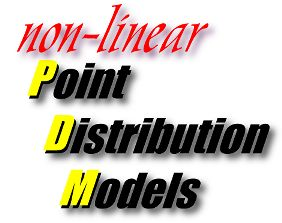Richard Bowden Vision  and VR Group Brunel University Uxbridge Middlesex UB8 3PH richard.bowden@brunel.ac.uk www.brunel.ac.uk/~emstrrb/ 29/10/98

Introduction to the Point Distribution Model

The principle behind the Point Distribution Model (PDM)  is that the shape and deformation of an object can be expressed statistically by formulating the shape as a vector representing a set of points that describe the object. This shape and its deformation (expressed with a training set, indicative of the object deformation) can then be learnt through statistical analysis. The same technique can be applied to more complex models of grey scale appearance or combinations of these techniques; however, the underlying linear mathematics for model representation remains the same.

To construct a simple PDM of a 2D contour, each pose of the model is described by a vector xe = (x1, y1, . . . ,xN ,yN), representing the set of points specifying the path of the contour. A training set of E vectors is then assembled for a particular model class. The training set E is then aligned (using translation, rotation and scaling) and the mean shape calculated by finding the average vector. To represent the deviation within the shape of the training set Principle Component Analysis is performed on the deviation of the example vectors from the mean using eigenvector decomposition on the covariance matrix S of E.

The t unit eigenvectors of S (corresponding to the t largest eigenvalues) supply the variation modes; t will generally be much smaller than N, thus giving a very compact model. A deformed shape x is generated by adding weighted combinations of vj to the mean shape :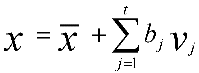where bj is the weighting for the jth variation vector. Suitable limits for bj are between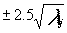and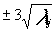, where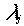is the jth largest eigenvalue of S.

This simple statistical analysis is possible due to the assumption that the training set forms a cluster in 2n dimensional space and that this cluster is hyper-elliptical in shape. Using this assumption, Principle Component Analysis (PCA) can be used to extract the centre and the bounds of the ellipse along each of its major axis by analysing the eigenvectors/values of the covariance matrix.

PCA projects the data into a linear subspace with a minimum loss of information by multiplying the data by the eigenvectors of the covariance matrix (S). By analysing the magnitude of the corresponding eigenvalues, the minimum dimensionality of the space on which the data lies can be calculated and the information loss estimated .

The principle is demonstrated in figure 1, where the primary orthogonal axis and its bounds are determined which describe the 3D elliptical cluster. The mean value at the centre of the cluster is the mean shape of the training set. The vector v1 is the primary axis of the cluster with v2, the secondary orthogonal axis and v3 the third. Once this analysis has been performed the shape can be restricted to lie within this cluster so constraining the shape of the model. From this model all shapes that were present in E can be reconstructed. In addition, many other shapes (hopefully viable) not present within the original training set can also be constructed.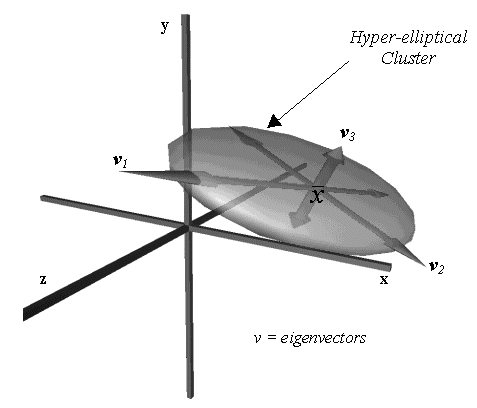Figure 1

Unfortunately, for all but the most simple of PDMs this hyper-elliptical assumption does not hold true and the linear mathematics behind the process generates a weak/un-robust model.

Why make the assumption of a linear model?

Working on the basis that similar shapes will produce similar vectors, it is a fair assumption that the training set will generate a cluster in space. However it is unfair to assume that this cluster will be uniform in shape and size. As more complex models are considered the training set may even generate multiple, separate clusters in the shape space.

These non-linear training sets produce complex high dimensional shapes which, when modelled through the linear mathematics of PCA, produce unreliable models.

Figure 2 illustrates this fact with a curved training set in two dimensions. The bounding box shows the limits of the model as constrained by linear PCA, with the extracted orthogonal linear axis shown in red. Using the standard linear formulation of the PDM, any point within the bounding box could be reconstructed. This results in an area which is over twice the size of that which would ideally be encompassed by the model. It should also be noted that the mean shape of the linear PDM (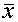) is not the mean point of the original curved cluster and the secondary mode of variation must be used to rectify this error. The blue arrows demonstrate how non-linear axis could be fitted to the data set to better model this shape. The primary mode of variation is now curved and closely follows the curvature of the data, the secondary modes change with respect to this primary curved axis.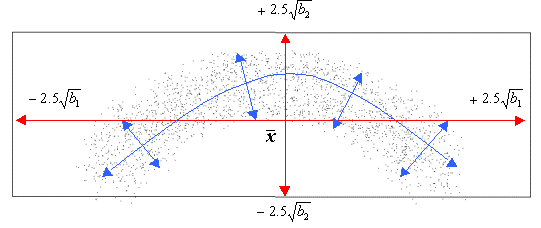Figure 2

What are the sources of non-linearity?

There are two basic types of non-linearity which are present within PDMs. There exists non-linearity that is the result of natural curvature of the model. This is present when key points of the model move in a non-linear fashion within the image frame, often pivotal rotations about some point. The second major source of non-linearity is introduced from the incorrect identification of landmark points during the assembly of the training set. These sources of non-linearity produce by far the worst non-linearity and often produce discontinues shape spaces that cannot be modelled by a single linear PDM.

Rotational Non-linearity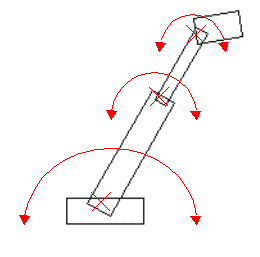Figure 3

Figure 3 demonstrates a model that contains pivotal rotation. A training set of examples are generated, demonstrating the rotational movement of the constituent components of the synthetic robot arm. After performing linear PCA to produce a PDM the resulting first two primary modes are shown in figure 4. The non-linearity of the model is clear in the distortion of the dimensions of the robot arm. The primary mode encompasses movement horizontally in the image plane, but also includes distortion in size, which must be rectified by other, higher modes of variation. The second mode encompasses movement in the vertical with more extreme distortions in size, especially at the head of the model. For shapes below the mean, along this second axis, the model deforms to shapes which are not representative of the training set by inverting the arm back upon itself.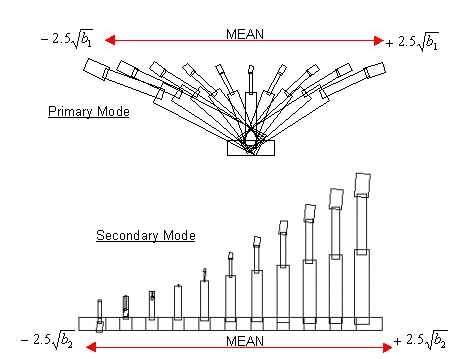Figure 4

High Order Non-linearity

Non-linearity introduced by the incorrect identification of landmark points introduces more complex non-linearity into the model. Consider the contour in figure 5. Using a simple scheme to allocate landmark points along the boundary, the contour is resampled to produce a consistent mathematical formulation for shape analysis. In this example, the highest point of the contour is located and marked as the black circle. Two further landmark points are then allocated at the mid-point between the highest point and the beginning of the contour (red), and the end of the contour (yellow).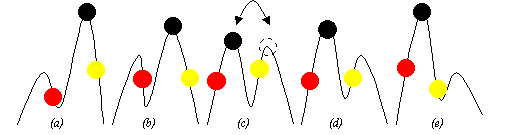Figure 5

As the contour changes its shape from a to b, the highest point remains consistent. However, as the contour changes from b to c, the highest point becomes a different part of the contour and this in turn affects the allocation of all the landmark points. As the contour continues through d and e this new labelling remains. Mathematically this manifests itself as two discontinues areas in the shape space. In addition to this, the simple mid-point assignment of subsequent landmarks shifts the landmarks along the boundary as it moves, producing curvature in shape space. This is demonstrated in figure 6 by showing what the resulting shape space may look like. It can also be seen that the mean shape does not exist in the training set due to the separate clusters and therefore, the principal modes do not represent the data accurately.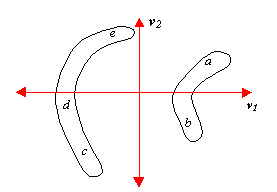Figure 6

How do we avoid non-linearity?

Rotational non-linearity

Where rotational non-linearity is known to be present within a model this can be removed/reduced by mapping the model into an alternative linear space.

Heap and Hogg suggested using a log polar mapping to remove non-linearity from the training set . This allows a non-linear training set to be projected into a linear space where PCA can be used to represent deformation. The model is then projected back into the original space. Although a useful suggestion for applications where the only non-linearity is pivotal and represented in the paths of the landmark points in the original model, it does not provide a solution for the high non-linearity generated from other sources.

High order non-linearity

By carefully selecting landmark points by hand, a near optimum labelling can be achieved which will minimise the non-linearity of a training set. However, for all but the most simple of cases this is not a feasible solution. Often semi-automated procedures are used where a user can speed up the process of labelling example shapes for analysis. Fully automated procedures are rarely used due to the problems of correctly assigning landmarks and the highly non-linear models that this produces.

It has been proposed by Kotcheff and Taylor that non-linearity introduced during assembly of a training set could be eliminated by automatically assigning landmark points in order to minimise the non-linearity of the corresponding training cluster . This can be estimated by analysing the size of the linear PDM that represents the training set. The more non-linear a proposed formulation of a training set, the larger the PDM needed to encompass the deformation. The procedure was demonstrated using a small test shape and scoring a particular assignment of landmark points according to the size of the training set (gained from analysis of the principle modes and the extent to which the model deforms along these modes, i.e. the eigenvalues of the covariance matrix). This was formulated as a minimisation problem, using a genetic algorithm. The approach performed well although at a heavy computation cost .

As the move to larger, more complex models or 3D models is considered, where dimensionality of the training set is high, this approach becomes unfeasible. A more generic solution is to use accurate non-linear representations.

How do we model non-linearity?

As linear PCA is used for linear PDMs, so, non-linear PCA can be used to model non-linear PDMs and many researchers have proposed approaches to this end.

Sozou et al first proposed using polynomial regression to fit high order polynomials to the non-linear axis of the training set . Although this compensates for some of the curvature represented within the training set, it does not adequately compensate for higher order non-linearity, which manifests itself in the smaller modes of variation as high frequency oscillations. In addition, the order of the polynomial to be used must be selected and the fitting process is time consuming.

Sozou et al further proposed modelling the non-linearity of the training set using a backpropagation neural network to perform non-linear principle component analysis . This performs well, however the architecture of the network is application specific; also, training times and the optimisation of network structure are time consuming.

What is required is a means of modelling the non-linearity accurately, but with the simplicity and speed of the linear model.

Several researchers have proposed alternatives, which utilise non-linear approximations, estimating non-linearity through the combination of multiple smaller linear models . These approaches have been shown to be powerful at modelling complex non-linearity in extremely high dimensional feature spaces .

The basic principle behind all these approaches is to break up any curvature into piecewise linear patches, which estimate the non-linearity rather than modelling it explicitly. This is akin to the polygonal representation of a surface. A smooth curved surface can be estimated by breaking it down into small linear patches. In the field of computer graphics this technique is performed to reduce render time. There exists, of course, a trade off between visual accuracy and computation speed (where the minimum numbers of polygons are used to achieve the desired appearance). The same problem is present in non-linear PDM estimation, where the minimum number of linear patches that accurately represent the model must be determined.

Bregler and Omohundro suggested modelling non-linear data sets of human lips using a Shape Space Constraint Surface. The surface constraints are introduced to the model by separating the space surface into linear patches using cluster analysis. However the dimensionality of these 'lip' shape spaces is low and and has low non-linearity due to the simplified application of the work.

Cootes and Taylor suggested modelling non-linear data sets using a gaussian mixture model, which is fitted to the data using Expectation Maximisation . Multiple gaussian clusters are fitted to the training set. This provides a more reliable model as constraints are placed upon the bounds of each piecewise patch of the shape space, which is modelled by the position, and size of each gaussian.

Both of these estimation techniques become unfeasible as dimensionality and training set size increase. However by projecting the training set down into the linear subspace as derived from PCA, the dimensionality and therefore computation complexity of the non-linear analysis can be reduced significantly to facilitate statistical and probabilistic analysis of the training set. This projection relies upon the dimensional reduction of PCA while retaining the preservation of the important information, the shape of the training set .

Bowden et al have shown how this technique can be used along with a fuzzy clustering algorithm to build composite models using linear patches to segregate the training set and estimate the non-linearity. It has also been shown how this technique demonstrates superior performance to other approaches in both accuracy and speed .

Bowden has also proposed that analysis of the path taken by the training set through the non-linear feature space can be used to provide a probabilistic model of how the model varies through shape space in addition to an invaluable aid to classification .

References

1. Bowden, R., PhD Thesis, In Preparation, Brunel University, UK.
2. Bowden, R., Mitchell, T. A., Sahardi, M., Cluster Based non-linear Principle Component Analysis, IEE Electronics Letters, 23rd Oct 1997, 33(22), pp1858-1858.
3. Bowden, R., Mitchell, T. A., Sahardi, M., Reconstructing 3D Pose and Motion from a Single Camera View.
4. Cootes T.F., Taylor C.J., A Mixture Model for Representing Shape Variation, In: Clark A F, ed. British Machine Vision Conference 1997, BMVC'97, University of Essex, UK: BMVA, 110-119.
5. Cootes TF, Taylor CJ, Cooper DH, Graham J. Active Shape Models - Their Training and Application. Computer Vision and Image Understanding 1995;61(1):38-59.
6. Heap T, Hogg D. Automated Pivot Location for the Cartesian-Polar Hybrid Point Distribution Model. In: Pycock D, ed. British Machine Vision Conference 1995, BMVC'95. University of Birmingham, Birmingham, UK: BMVA, 1995:97-106.
7. Heap T, Hogg D. Improving Specificity in PDMs using a Hierarchical Approach. In: Clark A F, ed. British Machine Vision Conference 1997, BMVC'97, University of Essex, UK: BMVA, 80-89.
8. Sozou PD, Cootes TF, Taylor CJ, Di-Mauro EC. A Non-linear Generalisation of PDMs using Polynomial Regression. In: Hancock E, ed. British Machine Vision Conference 1994, BMVC'94. University of York: BMVA Press, 1994:397-406.
9. Sozou PD, Cootes TF, Taylor CJ, Di Mauro EC. Non-Linear Point Distribution Modelling using a Multi-Layer Perceptron. In: Pycock D, ed. British Machine Vision Conference 1995, BMVC'95. University of Birmingham, Birmingham, UK: BMVA, 1995:107-116.
10. Kotcheff, A. C. W., Taylor, C. J., Automatic Construction of Eigenshape Models by Genetic Algorithms. In: Proc. International Conference on Information Processing in Medical Imaging 1997, Lecturenotes in Computer Science Issue 1230, 1997, Springer Verlag, pp1-14.
11. Bregler, C., Omohundro, S.: ‘Surface Learning with Applications to Lip Reading’, Advances in Neural Information Processing Systems 6, San Francisco, CA, 1994

Richard Bowden
Vision  and VR Group
Brunel University
Uxbridge
Middlesex
UB8 3PH
richard.bowden@brunel.ac.uk
29/10/98